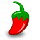# Plotting ROC Curves of Fingerprint Similarity¶

## Problem¶

You want to evaluate the performance of a molecule fingerprint method for discriminating between a set of molecules with the same activity class and a set of decoys. As the Drawing ROC Curve recipe shows that the performance of binary classification methods can be visualized (an evaluated) by depicting ROC curves and calculating AUC (area under the curve) number.

In order to perform cross validation for the fingerprint method, the generated plot (see Figure 1) depicts one ROC curve (thin green line) for each of the molecule from the activate set as well as the mean ROC curve (thick green line) and standard deviation (gray region). This graph along with the AUC number gives a clear picture about how well a fingerprint method works to identify molecules that are in the same activity class.

For more details about how to interpret a ROC curves and an AUC, see the Drawing ROC Curve recipe.Figure 1. Validation of Tree fingerprint method as binary classification for ACE inhibitors

## Ingredients¶

 numpy - Python for scientific computing matplotlib - Python 2D plotting library scikit-learn - Python for machine learning OEChem TK - cheminformatics toolkit GraphSim TK - fingerprinting toolkit

This recipe is based on the scikit-learn example: Receiver Operating Characteristic (ROC) with cross validation

## Difficulty level¶fprocs2img.py

## Solution¶

The plot_ROC_curves function calculates and depicts the ROC response for each molecule of the same activity class. Prior to calling the plot_ROC_curves function, two fingerprint databases are initialized with a specific fingerprint type (Tree, Path, Circular). The first, active_fpdb, stores the fingerprints of molecules that belong to the same activity class. While the other database, decoy_fpdb, stores fingerprints for decoy molecules i.e. molecules with different or unknown activity classes.

The plot_ROC_curves function iterates over all the fingerprint in the active dataset and calculates all the similarity scores for both the actives and the decoys by calling the OEFastFPDatabase.GetScores method (lines 19-20). The option (OEFPDatabaseOptions) used for calculating similarity scores ensures that all similarity scores are returned (lines 12-13). The calculated similarity scores are then combined and the ROC curve and AUC number are calculated (and accumulated).

After calculating (and depicting) all the ROC curves to see the variance of the curve for each molecule of the active set (thin green lines), the mean ROC curve (thick green line) and standard deviation (gray region) are also depicted. Finally, the mean AUC (area under curve) and its standard deviation are calculated and plotted.

The generated graph reveals how well the fingerprint method performs for the given activity class and how the retrial rate can be effected when different molecules from the active set are used to the other actives.

 1def plot_ROC_curves(plt, active_fpdb, decoy_fpdb):
2    """
3    Plots the ROC curves and calculate AUC
4
5    :type plt: matplotlib.pyplot
6    :type active_fpdb: oegraphsim.OEFastFPDatabase
7    :type decoy_fpdb: oegraphsim.OEFastFPDatabase
8    """
9
10    tprs = []
11    aucs = []
12    mean_fpr = linspace(0, 1, 100)
13
14    limit = 0  # zero means no limit all scores returned
15    opts = oegraphsim.OEFPDatabaseOptions(limit, oegraphsim.OESimMeasure_Tanimoto)
16    fp = oegraphsim.OEFingerPrint()
17    for fpidx in range(active_fpdb.NumFingerPrints()):
18        if not active_fpdb.GetFingerPrint(fp, fpidx):
19            continue
20
21        decoy_scores = [si.GetScore() for si in decoy_fpdb.GetScores(fp, opts)]
22        active_scores = [si.GetScore() for si in active_fpdb.GetScores(fp, opts)]
23
24        active_flags =  * len(decoy_scores) +  * len(active_scores)
25        scores = decoy_scores + active_scores
26        tpr, fpr, treshold = roc_curve(active_flags, scores)
27        tprs.append(interp(mean_fpr, fpr, tpr))
28        roc_auc = auc(fpr, tpr)
29        aucs.append(roc_auc)
30        plt.plot(fpr, tpr, color='green', linewidth=1, alpha=0.10, label=None)
31
32    mean_tpr = mean(tprs, axis=0)
33    mean_tpr[-1] = 1.0
34    mean_auc = auc(mean_fpr, mean_tpr)
35    std_auc = std(aucs)
36
37    plt.plot(mean_fpr, mean_tpr, color='green',
38             label=r'Mean ROC (AUC = %0.2f $\pm$ %0.2f)' % (mean_auc, std_auc),
39             linewidth=2.0, alpha=0.80)
40
41    std_tpr = std(tprs, axis=0)
42    tprs_upper = minimum(mean_tpr + std_tpr, 1)
43    tprs_lower = maximum(mean_tpr - std_tpr, 0)
44    plt.fill_between(mean_fpr, tprs_lower, tprs_upper, color='grey', alpha=0.33,
45                     label=r'$\pm$ 1 std. dev.')


## Usage¶

Usage

fprocs2img.py and two pre-generated binary fingerprint files: ACE-tree.fpbin and negative-tree.fpbin

The following command will generate the image shown in Figure 1.

prompt > python3 fprocs2img.py -active ACE-tree.fpbin -decoy negative-tree.fpbin fprocs.svg


The binary fingerprint files can be generated with the makefastfp.py script described in the Rapid Similarity Searching of Large Molecule Files recipe.

### Command Line Parameters¶

Simple parameter list
fingerprint database options
-memorytype : Fingerprint database memory type

input/output options
-active : Input filename of binary fingerprint database of actives
-decoy : Input filename of binary fingerprint database of decoys
-out : Output filename of the generated image


## Discussion¶

The Figure 1 reveals that a Tree fingerprint method, with very high AUC number (0.96), performs really well for ACE inhibitors of the Briem-Lessel validation set ([Briem-Lessel-2000]). In comparison, the same Tree fingerprint method performs poorly for the PAF Antagonist activity class in the same validation set (see Figure 2).Figure 2. Validation of Tree fingerprint method as binary classification for PAF Antagonist

The default parameters types (Tree, Path, and Circular) available in GraphSim TK are rigorous calibrated on the Briem-Lessel [Briem-Lessel-2000], Hert-Willett [Hert-Willett-2004], and Grant [Grant-2006] benchmark sets. GraphSim TK also provides facilities to construct user-defined fingerprints with the following adjustable parameters:

• the atom and bond typing that define which atom and bond properties are encoded into the fingerprints.

• the size of the fragments that are exhaustively enumerated during the fingerprint generation

• the size of the generated fingerprint (in bits)

However, an effective user-defined fingerprint can not be designed without understanding the effect of the different parameters on the overall performance.

The default fingerprint size used in GraphSim TK for Tree, Path, and Circular fingerprint types is 4096-bit long. By reducing the fingerprint size to 512-bits, the speed of the fingerprint search can accelerated about 8-fold, but not without reducing the power to discriminate between structurally similar and dissimilar molecules (see Table 1).